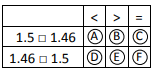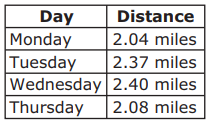# MAFS.4.NF.3.7Archived StandardExport Print
Compare two decimals to hundredths by reasoning about their size. Recognize that comparisons are valid only when the two decimals refer to the same whole. Record the results of comparisons with the symbols >, =, or <, and justify the conclusions, e.g., by using a visual model.

General Information
Subject Area: Mathematics
Domain-Subdomain: Number and Operations - Fractions
Cluster: Level 2: Basic Application of Skills & Concepts
Cluster: Understand decimal notation for fractions, and compare decimal fractions. (Major Cluster) -

Clusters should not be sorted from Major to Supporting and then taught in that order. To do so would strip the coherence of the mathematical ideas and miss the opportunity to enhance the major work of the grade with the supporting clusters.

Date of Last Rating: 02/14
Status: State Board Approved - Archived
Assessed: Yes
Test Item Specifications

• Assessment Limits :
Decimals may reference the same whole entity. Decimals are limited to tenths and hundredths. Decimals may be greater than 1. Items may not require a comparison of visual models in isolation.
• Calculator :

No

• Context :

Allowable

Sample Test Items (4)
• Test Item #: Sample Item 1
• Question: Mr. Shelby bought a new plant. The plant grew 2.6 centimeters in the first week and 3.42 centimeters the second week.

Select all the true comparisons of the plant growth for the two weeks.

• Difficulty: N/A
• Type: MS: Multiselect

• Test Item #: Sample Item 2
• Question: Zach and Karla each have seeds they will plant in a class garden. Zach’s seeds weigh 1.5 grams. Karla’s seeds weigh 1.46 grams.

Fill in the circles to select the correct symbol for each comparison.• Difficulty: N/A
• Type: MI: Matching Item

• Test Item #: Sample Item 3
• Question: Allison wrote down a decimal number that is greater than 0.58 but less than 0.62.

What is one number Allison could have written down?

• Difficulty: N/A
• Type: EE: Equation Editor

• Test Item #: Sample Item 4
• Question: The table shows the distances that Brianna ran on four days.Which comparison about the distances is true?

• Difficulty: N/A
• Type: MC: Multiple Choice

## Related Courses

This benchmark is part of these courses.
5012060: Mathematics - Grade Four (Specifically in versions: 2014 - 2015, 2015 - 2022, 2022 and beyond (current))
7712050: Access Mathematics Grade 4 (Specifically in versions: 2014 - 2015, 2015 - 2018, 2018 - 2022, 2022 and beyond (current))
5020110: STEM Lab Grade 4 (Specifically in versions: 2016 - 2022, 2022 and beyond (current))
5012065: Grade 4 Accelerated Mathematics (Specifically in versions: 2019 - 2022, 2022 and beyond (current))
5012015: Foundational Skills in Mathematics 3-5 (Specifically in versions: 2019 - 2022, 2022 and beyond (current))

## Related Access Points

Alternate version of this benchmark for students with significant cognitive disabilities.

## Related Resources

Vetted resources educators can use to teach the concepts and skills in this benchmark.

## Formative Assessments

Using Models to Compare Decimals:

Students are asked to compare decimals by drawing a visual model and record the comparison using the less than, greater than, or equal to symbol.

Type: Formative Assessment

Comparing Decimals in Context:

Students are asked to compare two pairs of decimals in the context of word problems and to record a comparison using an inequality symbol.

Type: Formative Assessment

Comparing Four Tenths:

Students are asked to consider two grids with different sized wholes and determine if both models show four-tenths.

Type: Formative Assessment

Compare Decimals:

Students are asked to compare four pairs of decimals using the less than, greater than, or equal to symbols.

Type: Formative Assessment

## Lesson Plans

Cell Phone Inquiry:

Students will determine what cell phone would be the best phone for their teacher to purchase for science class. Factors to consider are price, touch screen, camera, voice command, weight and display size. Students will need to compare decimals to determine how to order and rank the phone brands.

Type: Lesson Plan

Comparing and Ordering Decimals:

In this cooperative learning activity, students will have five sets of decimal cards to sort and put in order - least to greatest. The lesson starts with a short whole group activity and then breaks off in to structured groups. The teacher is free to interact with each of the groups and monitor progress, participation, and understanding.

Type: Lesson Plan

Students will help Amazing Alice Cookies choose the perfect chocolate chip brand to use for their cookies. Students will be given data in the form of fractions and decimals. Fourth grade students will compare decimals and order and compare fractions. Students will write a letter describing their procedure to the client.

Type: Lesson Plan

## Original Student Tutorial

Learn how to locate decimals on a number line and compare decimals to save the Decis from a wizard's spell in this interactive tutorial.

Type: Original Student Tutorial

Using Place Value:

Each part of this task highlights a slightly different aspect of place value as it relates to decimal notation. More than simply being comfortable with decimal notation, the point is for students to be able to move fluidly between and among the different ways that a single value can be represented and to understand the relative size of the numbers in each place.

## Tutorial

Comparing Two Decimals with a Visual Model:

In this Khan Academy tutorial video two decimals are compared using grid diagrams.

Type: Tutorial

## Virtual Manipulative

Fraction Models:

An interactive tool to represent a fraction circle, rectangle, or set model with numerators and denominators ranging from 1 to 100. The decimal and percent equivalents of the created fraction are also displayed.

Type: Virtual Manipulative

## STEM Lessons - Model Eliciting Activity

Students will help Amazing Alice Cookies choose the perfect chocolate chip brand to use for their cookies. Students will be given data in the form of fractions and decimals. Fourth grade students will compare decimals and order and compare fractions. Students will write a letter describing their procedure to the client.

Cell Phone Inquiry:

Students will determine what cell phone would be the best phone for their teacher to purchase for science class. Factors to consider are price, touch screen, camera, voice command, weight and display size. Students will need to compare decimals to determine how to order and rank the phone brands.

## MFAS Formative Assessments

Compare Decimals:

Students are asked to compare four pairs of decimals using the less than, greater than, or equal to symbols.

Comparing Decimals in Context:

Students are asked to compare two pairs of decimals in the context of word problems and to record a comparison using an inequality symbol.

Comparing Four Tenths:

Students are asked to consider two grids with different sized wholes and determine if both models show four-tenths.

Using Models to Compare Decimals:

Students are asked to compare decimals by drawing a visual model and record the comparison using the less than, greater than, or equal to symbol.

## Original Student Tutorials Mathematics - Grades K-5

Learn how to locate decimals on a number line and compare decimals to save the Decis from a wizard's spell in this interactive tutorial.

## Student Resources

Vetted resources students can use to learn the concepts and skills in this benchmark.

## Original Student Tutorial

Learn how to locate decimals on a number line and compare decimals to save the Decis from a wizard's spell in this interactive tutorial.

Type: Original Student Tutorial

Using Place Value:

Each part of this task highlights a slightly different aspect of place value as it relates to decimal notation. More than simply being comfortable with decimal notation, the point is for students to be able to move fluidly between and among the different ways that a single value can be represented and to understand the relative size of the numbers in each place.

## Tutorial

Comparing Two Decimals with a Visual Model:

In this Khan Academy tutorial video two decimals are compared using grid diagrams.

Type: Tutorial

## Parent Resources

Vetted resources caregivers can use to help students learn the concepts and skills in this benchmark.